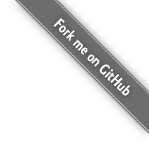Top Close Open

SYNOPSIS

Top Close Open
[% USE Math %]

[% Math.sqrt(9) %]

DESCRIPTION

Top Close Open

The Math plugin provides numerous mathematical functions for use within templates.

METHODS

Top Close Open

Template::Plugin::Math makes available the following functions from the Perl core:

• abs
• atan2
• cos
• exp
• hex
• int
• log
• oct
• rand
• sin
• sqrt
• srand

In addition, if the Math::Trig module can be loaded, the following functions are also available:

• pi
• tan
• csc
• cosec
• sec
• cot
• cotan
• asin
• acos
• atan
• acsc
• acosec
• asec
• acot
• acotan
• sinh
• cosh
• tanh
• csch
• cosech
• sech
• coth
• cotanh
• asinh
• acosh
• atanh
• acsch
• acosech
• asech
• acoth
• acotanh

If the Math::TrulyRandom module is available, and you've got the time to wait, the truly_random_number method is available:

[% Math.truly_random_number %]

AUTHOR

Top Close Open

Andy Wardley <abw@wardley.org> http://wardley.org/